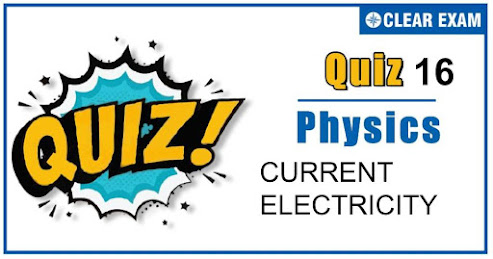## [LATEST]\$type=sticky\$show=home\$rm=0\$va=0\$count=4\$va=0

As per analysis for previous years, it has been observed that students preparing for NEET find Physics out of all the sections to be complex to handle and the majority of them are not able to comprehend the reason behind it. This problem arises especially because these aspirants appearing for the examination are more inclined to have a keen interest in Biology due to their medical background. Furthermore, sections such as Physics are dominantly based on theories, laws, numerical in comparison to a section of Biology which is more of fact-based, life sciences, and includes substantial explanations. By using the table given below, you easily and directly access to the topics and respective links of MCQs. Moreover, to make learning smooth and efficient, all the questions come with their supportive solutions to make utilization of time even more productive. Students will be covered for all their studies as the topics are available from basics to even the most advanced. .

Q1. In the given figure, battery E is balanced on 55 cm length of potentiometer wire but when a resistance of 10 Ω is connected in parallel with the battery then it balances on 50 cm length of the potentiometer wire then internal resistance r of the battery is?
•  1 Ω
•  3 Ω
•  10 Ω
•  5 Ω
Solution
r=((l1-l2)/l2 )×R'⇒r=((55-50)/50)×10=1Ω

Q2. Which statement is true? (i) Kirchoff’s law is equally applicable to both AC and DC. (ii) Semiconductors have a positive temperature coefficient of resistance. (iii) Meter bridge is greater sensitive when the resistance of all four arms of the bridge is of the same order. (iv) The emf of a cell depends upon the size and area of electrodes.
•  (i) and (iv)
•  (ii) and (iv)
•  (iii) and (iv)
•  None of these
Solution
In a potentiometer there is no current drawn from the cell whose emf is to be measured whereas a voltmeter always draws some current from the cell. Hence, the emf of a cell can be measured accurately using a potentiometer.

Q3. An electric iron draws 5 amp, a TV set draws 3 amp and refrigerator graws 2 amp from a 220 volt main line. The three appliances are connected in parallel. If all the three are operating at the same time, the fuse used may be of?
•  20 amp
•  5 amp
•  15 amp
•  10 amp
Solution
Current capacity of a fuse wire should be slightly greater than the total rated load current

Q4. The ratio of the amounts of heat developed in the four arms of a balanced Wheatstone bridge, when the arms have resistance P=100 Ω; Q=10 Ω;R=300 Ω and S=30 Ω respectively is?
•  3 : 30 : 1 : 10
•  30 : 3 : 10 :1
•  30 : 10 : 1 : 3
•  30 : 1 : 3 : 10
Solution
Let I be the total current passing through balanced wheat stone bridge. Current through arms of resistances P and Q in series is I1=(I×300)/(330+110)=3/4 I and current through arms of Resistances R and S in series is I2=(I×110)/(330+110)=1/4 I ∴ Ratio of heat developed per sec HP ∶HQ ∶HR ∶HS =(3/4 I)2×100∶(3/4 I)2×10∶(1/4 I)2×300∶(1/4 I)2×30 =30∶3∶10∶1.

Q5. Length of a hollow tube is 5m, it’s outer diameter is 10 cm and thickness of it’s wall is 5 mm. If resistivity of the material of the tube is 1.7×10-8 Ω×m then resistance of tube will be?
•  5.6×10-5 Ω
•  2×10-5 Ω
•  4×10-5 Ω
•  None of these
Solution
By using R=ρ.l/A;here A=π(r22-r12 ) Outer radius r2=5cm Inner radius r1=5-0.5=4.5 cm
So R=1.7×10-8×5/(π{(5×10-2 )2-(4.5×10-2 )2}) =5.6×10-5 Ω

Q6. A milliammeter of range 0-30mA has internal resistance of 20 Ω. The resistance to be connected in series to convert it into a voltmeter of maximum reading 3V is?
•  49 Ω
•  80 Ω
•  40 Ω
•  30 Ω
Solution
R= V/Ig -G=3/(30×10-3 )-20=102-20=80Ω

Q7. A lamp having tungsten filament consumes 50 W. Assume the temperature coefficient of resistance for tungsten is 4.5×10-3-1 and temperature of the surrounding is 20℃. When the lamp burns, the temperature of its filament becomes 2500℃ , then the power consumed at the moment switch is on, is?
•  608 W
•  710 W
•  215 W
•  580 W
Solution
Let R0 = resistance of filament at room temperature Rt = resistance of filament at 2500℃ Similarly powers, P0 and Pt. Here, voltage remains the same. P0=V2/R0 or R0=V2/P0 , Rt=V2/Pt also Rt=R0 [1+α(2500-20) ] and P0=Pt [1+α(2500-20)] =50[1+4.5×10-3 (2500-20)] = 608W

Q8. A heater coil cut into two equal parts and one part is connected with heater. Now heat generated in heater will be?
•  Twice
•  Half
•  One-fourth
•  Four times
Solution
Half

Q9. A long straight wire of a circular cross section (radius a) carries a steady current I and the current I is uniformly distributed across this cross-section. Which of the following plots represents the variation of magnitude of magnetic field B with distance r from the centre of the wire?
Solution
Magnetic field due to a long straight wire of radius a carrying current I at a point distant r from the centre of the wire is given as follows B=(μ0 Ir)/(2πa2 ) for r less than a B=(μ0 I)/2πr for r=a B=(μ0 I)/2πr for r>a The variation of magnetic field B with distance r from the centre of wire is shown in the figure

Q10. The resistance of a discharge tube is?
•  Ohmic
•  Non-ohmic
•  Both (a) and (b)
•  Zero
Solution
This is because of secondary ionisation which is possible in the gas filled in it## Want to know more

Please fill in the details below:

## Latest NEET Articles\$type=three\$c=3\$author=hide\$comment=hide\$rm=hide\$date=hide\$snippet=hide

Name

ltr
item
BEST NEET COACHING CENTER | BEST IIT JEE COACHING INSTITUTE | BEST NEET, IIT JEE COACHING INSTITUTE: Current Electricity Quiz 16
Current Electricity Quiz 16